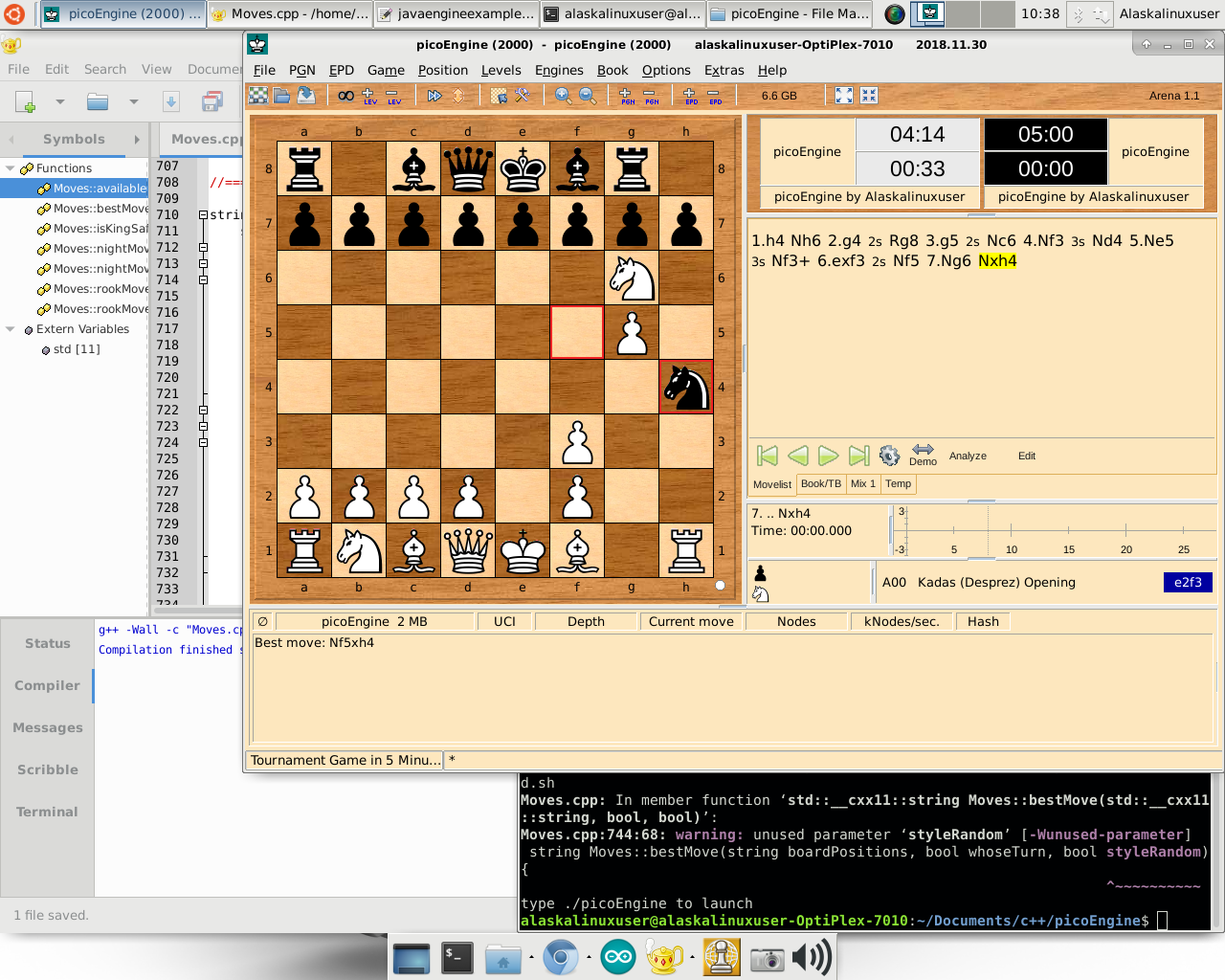##### Now the rooks can move too!

While it is only a small improvement, the rooks can now also be moved by picoEngine, which is a great step forward. Overall, that leaves 2 of 6 pieces complete for motion, or about 33% complete. Technically, when that is done, the engine will be completely playable, however, much work will be needed to make it “intelligent”.

While there are probably better ways to do this, I followed a pretty simple pattern for making the rook moves:

// Up moves
bool notI = true;
int j = 1;
int vert = 8;
int k = i;
if (i < 56) {
k = i + (vert * j);
}
while (theBoard[k] == ‘-‘ && notI) {
theseMoves.push_back(k);
vert += 8;
if (k < 56) {
k = i + (vert * j);
} else {
notI = false;
}
} // While it’s empty.
if (isupper(theBoard[k])) {
theseMoves.push_back(k);
} // When there is an enemy.

// Down moves
notI = true;
j = -1;
vert = 8;
k = i;
if (i > 7) {
k = i + (vert * j);
}
while (theBoard[k] == ‘-‘ && notI) {
theseMoves.push_back(k);
vert += 8;
if (k >7) {
k = i + (vert * j);
} else {
notI = false;
}
} // While it’s empty.
if (isupper(theBoard[k])) {
theseMoves.push_back(k);
} // When there is an enemy.

That is for “up” moves, and the pattern repeats for down, left, and right. As always, you can check out the full commit on my GitLab.

Linux – keep it simple.